$$\newcommand{\Rv}{\mathbf{R}} \newcommand{\rv}{\mathbf{r}} \newcommand{\Qv}{\mathbf{Q}} \newcommand{\Av}{\mathbf{A}} \newcommand{\Aiv}{\mathbf{Ai}} \newcommand{\av}{\mathbf{a}} \newcommand{\xv}{\mathbf{x}} \newcommand{\Xv}{\mathbf{X}} \newcommand{\Kv}{\mathbf{K}} \newcommand{\yv}{\mathbf{y}} \newcommand{\Yv}{\mathbf{Y}} \newcommand{\zv}{\mathbf{z}} \newcommand{\alphav}{\mathbf{\alpha}} \newcommand{\Wv}{\mathbf{W}} \newcommand{\wv}{\mathbf{w}} \newcommand{\betav}{\mathbf{\beta}} \newcommand{\gv}{\mathbf{g}} \newcommand{\Hv}{\mathbf{H}} \newcommand{\dv}{\mathbf{d}} \newcommand{\Vv}{\mathbf{V}} \newcommand{\vv}{\mathbf{v}} \newcommand{\Uv}{\mathbf{U}} \newcommand{\uv}{\mathbf{u}} \newcommand{\tv}{\mathbf{t}} \newcommand{\Tv}{\mathbf{T}} \newcommand{\TDv}{\mathbf{TD}} \newcommand{\Tiv}{\mathbf{Ti}} \newcommand{\Sv}{\mathbf{S}} \newcommand{\Gv}{\mathbf{G}} \newcommand{\zv}{\mathbf{z}} \newcommand{\Zv}{\mathbf{Z}} \newcommand{\Norm}{\mathcal{N}} \newcommand{\muv}{\boldsymbol{\mu}} \newcommand{\sigmav}{\boldsymbol{\sigma}} \newcommand{\phiv}{\boldsymbol{\phi}} \newcommand{\Phiv}{\boldsymbol{\Phi}} \newcommand{\Sigmav}{\boldsymbol{\Sigma}} \newcommand{\Lambdav}{\boldsymbol{\Lambda}} \newcommand{\half}{\frac{1}{2}} \newcommand{\argmax}{\underset{#1}{\operatorname{argmax}}} \newcommand{\argmin}{\underset{#1}{\operatorname{argmin}}} \newcommand{\dimensionbar}{\underset{#1}{\operatorname{|}}} \newcommand{\grad}{\mathbf{\nabla}} \newcommand{\ebx}{e^{\betav_{#1}^T \xv_n}} \newcommand{\eby}{e^{y_{n,#1}}} \newcommand{\Tiv}{\mathbf{Ti}} \newcommand{\Fv}{\mathbf{F}} \newcommand{\ones}{\mathbf{1}_{#1}}$$

# Machine Learning for Brain-Computer Interfaces¶

## What are Brain-Computer Interfaces (BCI)?¶

Our brain determines what we think and what we do by controlling our movements. If our usual movements become restrited, due to injury or neurodegenerative diseases, can we directly measure brain activity to restore lost movements?

Two approaches to this have been developed:

Work at CSU on noninvasive BCI has used pie-menus for selection of choices, to select music and to control a mobile robot

## Using CSU's data for machine learning experiments¶

Visit our project's web site. From the Data web page download some data. Let's download Subject 11's data recorded with the g.GAMMAsys EEG system, and unzip the file eeg.zip to get s11-gammasys-home-impaired.json.zip, then unzip this file.

Now, read the json file.

In :
import numpy as np
import matplotlib.pyplot as plt
%matplotlib inline

In :
import zipfile
import json

In :
data = json.load(open('s11-gammasys-home-impaired.json','r'))

In :
len(data)

Out:
8
In :
data.keys()

Out:
dict_keys(['protocol', 'sample rate', 'notes', 'channels', 'device', 'location', 'date', 'eeg', 'impairment', 'subject'])
In :
data['protocol']

Out:
'3minutes'
In :
[d['protocol'] for d in data]

Out:
['3minutes',
'grid-p',
'grid-b',
'grid-d',
'letter-p',
'letter-b',
'letter-d',
'mentaltasks']
In :
letterb = data

In :
letterb['protocol'],letterb['sample rate'],letterb['notes'],letterb['subject'],letterb['date']

Out:
('letter-b', 256, '', 11, [2011, 6, 3])
In :
letterb['location'],letterb['impairment'],letterb['device'],letterb['channels']

Out:
('home',
'spinal',
'GAMMAsys',
['F3', 'F4', 'C3', 'C4', 'P3', 'P4', 'O1', 'O2'])
In :
eeg = letterb['eeg']

In :
eeg.keys()

Out:
dict_keys(['trial 1'])
In :
eeg = eeg['trial 1']
len(eeg)

Out:
9
In :
eeg = np.array(eeg)
eeg.shape

Out:
(9, 17691)
In :
eeg = eeg.T

In :
plt.plot(eeg);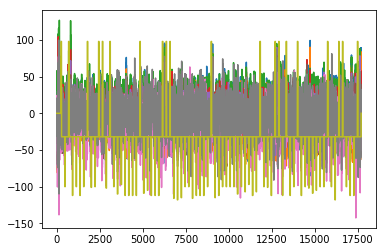In :
plt.plot(eeg[2000:2300,:]);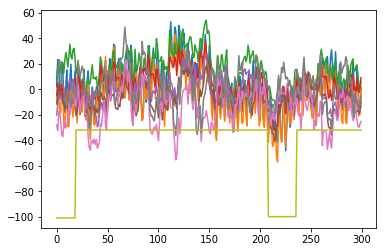The stimulus channel has the values

In :
np.unique(eeg[:,-1])

Out:
array([-118., -116., -115., -112., -110., -109., -107., -105., -102.,
-101., -100.,  -97.,  -32.,    0.,   98.])

Its value is 0 only before the trial starts. Of the non-zero values, only one is positive. This represents the letter to be counted—the target letter. The negative values are nontarget letters, except for -32, which is the ASCII code for a blank representing the times when no letter is displayed and the computer screen is black. The ASCII codes for all stimulus channel values can be converted to letters by

In :
[chr(int(n)) for n in np.abs(np.unique(eeg[:,-1]))]

Out:
['v', 't', 's', 'p', 'n', 'm', 'k', 'i', 'f', 'e', 'd', 'a', ' ', '\x00', 'b']

To investigate the presence or absence of P300 waves, we must segment the data into time windows following each stimulus presentation. First, let’s take a closer look. Plot just the data from the stimulus channel (index 8) and Channel P3 (index 5).

In :
plt.plot(eeg[3000:4000,[5,8]]);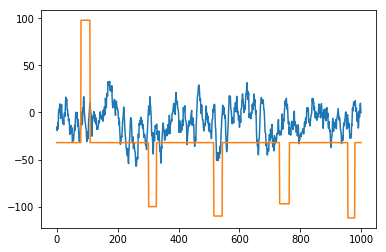It appears that a P300 wave is present following the one positive stimuli. Remember that this is the target letter ‘b’. The positive wave appears roughly 100 samples after the stimulus onset. Since the sampling frequency is 256 Hz, this is about 0.4 seconds, or 400 milliseconds, after the stimulus.

In :
100 / letterb['sample rate']

Out:
0.390625

Now let’s collect all of the segments from this trial. First, find the sample indices where each stimulus starts, collect the displayed letters for each stimulus, and keep track of which stimuli are the target letter.

In :
starts = np.where(np.diff(np.abs(eeg[:,-1])) > 0)
stimuli = [chr(int(n)) for n in np.abs(eeg[starts+1,-1])]
targetLetter = letterb['protocol'][-1]
targetSegments = np.array(stimuli) == targetLetter
len(starts),len(stimuli),len(targetSegments)

Out:
(80, 80, 80)
In :
for i,(s,stim,targ) in enumerate(zip(starts,stimuli,targetSegments)):
print(s,stim,targ,'; ',end='')
if (i+1) % 5 == 0:
print()

247 b True ; 461 d False ; 679 b True ; 903 p False ; 1119 d False ;
1334 p False ; 1555 d False ; 1771 b True ; 1987 e False ; 2207 d False ;
2423 b True ; 2642 b True ; 2863 f False ; 3079 b True ; 3301 d False ;
3515 n False ; 3731 a False ; 3955 p False ; 4171 p False ; 4391 p False ;
4610 p False ; 4827 b True ; 5039 m False ; 5262 i False ; 5475 p False ;
5698 p False ; 5911 p False ; 6130 b True ; 6354 b True ; 6567 b True ;
6790 t False ; 7003 v False ; 7222 s False ; 7439 d False ; 7659 d False ;
7875 t False ; 8095 p False ; 8311 p False ; 8529 p False ; 8751 d False ;
8966 b True ; 9187 s False ; 9401 p False ; 9619 d False ; 9841 d False ;
10059 d False ; 10279 d False ; 10491 n False ; 10715 d False ; 10931 k False ;
11146 a False ; 11363 p False ; 11587 k False ; 11799 b True ; 12022 d False ;
12239 e False ; 12454 p False ; 12678 b True ; 12894 b True ; 13110 p False ;
13326 b True ; 13547 p False ; 13763 d False ; 13979 b True ; 14199 p False ;
14419 d False ; 14635 f False ; 14855 m False ; 15073 i False ; 15291 d False ;
15507 d False ; 15729 b True ; 15947 d False ; 16163 v False ; 16382 b True ;
16599 b True ; 16819 d False ; 17031 p False ; 17255 p False ; 17467 b True ;


Good. Now, how long should each time window be? The number of samples between each stimulus onset is

In :
np.diff(starts)

Out:
array([214, 218, 224, 216, 215, 221, 216, 216, 220, 216, 219, 221, 216,
222, 214, 216, 224, 216, 220, 219, 217, 212, 223, 213, 223, 213,
219, 224, 213, 223, 213, 219, 217, 220, 216, 220, 216, 218, 222,
215, 221, 214, 218, 222, 218, 220, 212, 224, 216, 215, 217, 224,
212, 223, 217, 215, 224, 216, 216, 216, 221, 216, 216, 220, 220,
216, 220, 218, 218, 216, 222, 218, 216, 219, 217, 220, 212, 224,
212])

and the minimum number is

In :
np.min(np.diff(starts))

Out:
212

so let’s use time windows of 212 samples. We can build a matrix with rows being segments, and each row being all indices for that segment by doing this.

In :
indices = np.array([ np.arange(s,s+212) for s in starts ])
indices.shape

Out:
(80, 212)

And, finally, we can build an 80 x 212 x 8 array of segments.

In :
segments = eeg[indices,:8]
segments.shape

Out:
(80, 212, 8)

Typically, segments are averaged to minimize the effect of variations across multiple stimuli and, hopefully, to emphasize the parts of the signal that are common to the stimuli. Let’s average all of the target segments and all of the nontarget segments for the P4 channel (index 5), and plot two resulting mean segments.

In :
segments[targetSegments,:,5].shape

Out:
(20, 212)
In :
np.sum(targetSegments),np.sum(targetSegments==False)

Out:
(20, 60)
In :
plt.figure(figsize=(10,10))
targetMean = np.mean(segments[targetSegments,:,5],axis=0)
nontargetMean = np.mean(segments[targetSegments==False,:,5],axis=0)
xs = np.arange(len(targetMean)) / 256.0 * 1000  # milliseconds
plt.plot(xs, np.vstack((targetMean,nontargetMean)).T)
plt.legend(('Target','Nontarget'))
plt.xlabel('Milliseconds After Stimulus')
plt.ylabel('Mean Voltage ($\mu$ V)');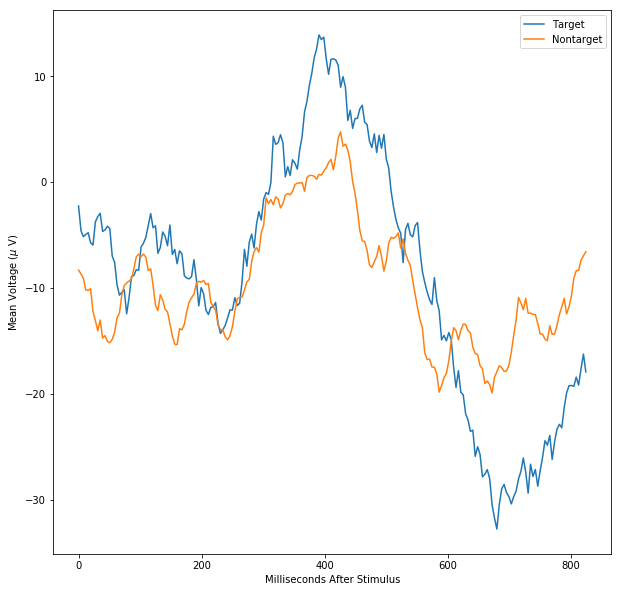Now, let's try to classify the segments.

In :
import qdalda as ql

In :
X = segments[:,:,5] # using channel 6
T = targetSegments.astype(int).reshape((-1,1))
X.shape,T.shape

Out:
((80, 212), (80, 1))
In :
for i in range(X.shape):
if T[i] == 1:
plt.plot(X[i,:],color='green')
else:
plt.plot(X[i,:],color='red')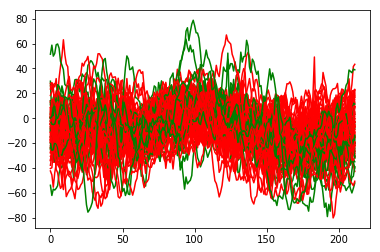In :
lda = ql.LDA()
lda.train(X,T)
predicted,probs,discs = lda.use(X)

In :
np.sum(predicted == T) / T.shape * 100

Out:
97.5
In :
plt.figure(figsize=(8,10))
plt.subplot(2,1,1)
plt.plot(T, 'o-')
plt.plot(predicted+0.1, 'o-')
plt.ylim(-0.1,1.2)
plt.subplot(2,1,2)
plt.plot(probs[:,1])
plt.ylabel('Clas Probabilities')

Out:
Text(0, 0.5, 'Clas Probabilities')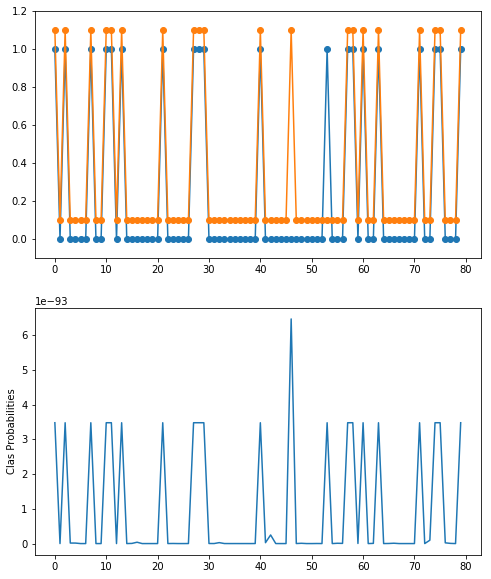But, what is wrong with this? Can we expect 97% correct on additional data?

In [ ]: